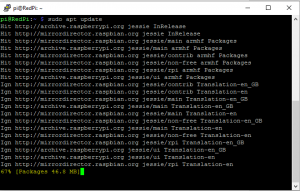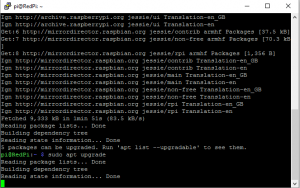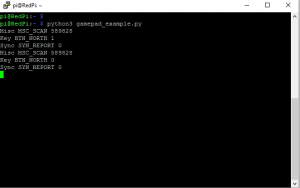# Python library ‘inputs’

Pi Wars is getting ever closer and I still have loads to do! One of the things I want to do was to get away from using the Pygame Python library for Joypad control, for a lighter weight solution and after searching around the web found Inputs. Inputs is a Python library by Zeth and is available for both Python 2 and 3. Inputs can handle inputs from HID devices, like keyboards, mice and joypads, for this post we will be just looking at how it works with gamepads. More details about inputs can be found at the project’s github page https://github.com/zeth/inputs

The testing for this post has been carried out on a clean install of the latest version of Raspbian.

we need to install Inputs, the easy way is to use pip or pip3 for Python3.

Before running pip, it is best to update your install of Raspbian

At the command line enter
``` sudo apt update ```
when completed enter
``` sudo apt upgrade ```
you may need to accepting any changes by typing

next you need to install the library itself by entering for Python 2.7
``` sudo pip install inputs ```
or for Python 3
``` sudo pip3 install inputs ```
the above steps should look like thisif you have any trouble with the above check your spelling and make sure you are connected to the internet!

Next we need to download a program to test your gamepad and find out the names of the buttons and axis. Enter the following at the command line

and run the program by entering
``` python3 gamepad_example.py ```
you should get a result like the following, and the output from a gamepad, the screenshot shows the triangle button on my controller being pressed and released.## Some examples

get_gamepad collects current status of the game controller into a list.

you can extract the information by using a ‘for’ loop,  for example the following code prints Backwards when the event code is for the left joystick and it’s status is is above 130.

```from inputs import get_gamepad
while True:
for event in events:
if event.code == "ABS_Y":
if event.state > 130:
print("Backwards")
```

the next example is for the left trigger, a button has two states pressed or released and is represented by 1 and 0 therefore can be tested with Boolean logic.

```from inputs import get_gamepad
while True:
for event in events:
if event.code == "BTN_TL":
if event.state:
print("Turbo")
else:
print("Turbo released")
```

For the Rock Candy controllers, I have written a skeleton code which you can base your code on for your robot or project. you can download the code to your Raspberry Pi by entering the following at the command line
```wget https://raw.githubusercontent.com/Corteil/blog_code/master/InputsTest.py ```
or you can type out the following

```#!/usr/bin/env python

# coding: Latin-1

# Load library functions we want

try:

print('Press CTRL+C to quit')

# Loop indefinitely

while True:

for event in events:

print(event.code, event.state)

if event.code == "ABS_Y":

if event.state > 130:

print("Backwards")

elif event.state < 125:

print("Forward")

if event.code == "ABS_Z":

if event.state > 130:

print("Right")

elif event.state < 125:

print("Left")

if event.code == "BTN_TL":

if event.state == True:

print("Turbo")

if event.code == "BTN_TR":

if event.state == True:

print("Tank")

if event.code == "BTN_NORTH":

if event.state == True:

print("Triangle")

if event.code == "BTN_SOUTH":

if event.state == True:

print("Square")

if event.code == "BTN_EAST":

if event.state == True:

print("Cross")

if event.code == "BTN_C":

if event.state == True:

print("Circle")

if event.code == "BTN_TR2":

if event.state == True:

print("Start")

if event.code == "BTN_TL2":

if event.state == True:

print("Select")

if event.code == "ABS_HAT0Y":

if event.state == -1:

elif event.state == 1:

if event.code == "ABS_HAT0X":

if event.state == -1:

elif event.state == 1:

if event.code == "BTN_WEST":

if event.state == True:

print("Top left")

if event.code == "BTN_Z":

if event.state == True:

print("Top right")

print("#### End ####")

# print(event.ev_type, event.code, event.state)

except KeyboardInterrupt:

# CTRL+C exit, disable all drives

print("stop")

print("bye")
```